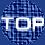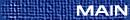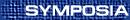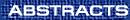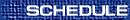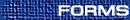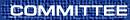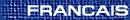Number Theory / Théorie des nombres
(A. Akbary and O. Kihel, Organizers)

E. BENJAMIN, Maine
To be announced

D. BRADLEY, Department of Mathematics and Statistics, University of Maine, Orono, Maine  04469-5752, USA
Shuffles and multiple zeta values

Multiple zeta values are values of multiple polylogarithms at positive integer arguments. They can also be viewed as a multiply nested sum extension of the Riemann zeta function. As noted by Pierre Cartier in his recent Bull. Amer. Math. Soc. article commemorating the 40th anniversary of the IHES, classifying the relations amongst multiple zeta values connects with some of the deepest problems in transcendental number theory. It is well-known that multiple zeta values satisfy certain ``shuffle'' relations. We describe here a new technique for proving certain shuffle convolution formulae. Applications include a short proof of a former conjecture of Zagier and some new results for multiple zeta values as well.

S. CHOI, Simon Fraser University, Burnaby, British Columbia
Norm of Littlewood polynomials

We are interested in studying the norm of Littlewood polynomials, i.e., polynomials with coefficients +1 or -1. The problem is to find polynomials having small L4 norms over the unit circle. The best known polynomials are Turyn polynomials whose coefficients are the shifted legendre symbols. Some old and new results about this problem will be discussed in this talk, especially for polynomials constructed by cyclic difference sets (Turyn polynomial is one of the examples).

A. COJOCARU, Department of Mathematics and Statistics, Queen's University, Kingston, Ontario  K7L 3N6
Square-free orders for non-CM elliptic curves modulo p

Let E be an elliptic curve defined over Q and without complex multiplication. We consider the problem of determining an effective asymptotic formula for the number of primes p < x of good reduction for E and such that the group of points of E modulo p has square-free order. This problem is related to the one regarding the cyclicity of E modulo p, but more challenging. The situation of an elliptic curve with complex multiplication can also be considered, however it is very different from the non-complex multiplication case and so has to be discussed on a different occasion.

CLIFTON CUNNINGHAM, University of Calgary, Calgary, Alberta
The geometry of depth-zero representations

It has been observed in a number of cases that the number of points on certain hyper elliptic curves over a finite field may be recovered as the values of depth zero representations of p-adic groups, but the nature of this relationship remains a mystery. In this talk I will describe a new geometric approach to these representations which promises to shed light on this and other related problems.

HENRI DARMON, McGill
To be announced

CHANTAL DAVID, Concordia
To be announced

L. DAVISON, Laurentian University
Levy constants for classes of continued fractions

Suppose (un)n ³ 1 is a bounded sequence of positive integers and a = [0,u1,u2,...,un¼] is the corresponding continued fraction with convergents ([(pn)/(qn)])n ³ 0. If the infinite word u = u1,u2,... has the property that any finite subword of u occurs with a frequency, then we prove that limn®¥[1/(n)]lnqn exists. An application of this result is given.

JEAN-MARIE DE KONINCK, Université de Laval, Québec  G1K 7P4
The median value of the k-th prime factor of an integer

Let p1(n) < p2(n) < ¼ be the distinct prime factors of an integer n ³ 2. Given a positive integer k, we investigate the distribution function of the arithmetic function n® pk(n). As an application of our result, we prove the estimate loglogp*k = k-c + O(1/Ök), where p*k stands for the median value of the k-th prime factor of an integer and where c=[1/3] +g-ån ³ 2 åp [1/(npn )] » 0.59483. This is joint work with Professor Gérald Tenenbaum.

SAÏD EL MORCHID, Casablanca
Base des unités cyclotomiques de certains composés de trois corps abéliens

On considère trois extensions ab\' eliennes réelles finies de Q, k1, k2 et k3 de conducteurs respectifs p1e1, p2e2 et p3e3, avec p1, p2 et p3 des nombres premiers distinsts deux à deux et e1, e2 et e3 des entiers naturels non nuls. L'objet de cette communication est de déterminer une base du groupe des unités cyclotomiques du composé k=k1k2k3. Ceci est un travail conjoint avec Hugo Chapdelaine.

J.G. HUARD, Canisius College, Buffalo, New York  14208-1098, USA
Sums of twelve and sixteen squares

For a positive integer k, let rk(n) denote the number of representations of the positive integer n as the sum of k squares. In 1987, using the theory of modular functions, Ewell proved a formula for r16(n) in terms of real-divisor functions and established a result for r12(n). In 1996, Milne obtained formulas for rk(n) where k is any integer of the form 4m2 or 4m(m+1). Milne combined methods from the theory of elliptic functions, continued fractions, hypergeometric functions, Schur functions, and Hankel and Tur án determinants. Using a recent elementary arithmetic identity of Huard, Ou, Spearman and Williams, we give elementary proofs of Ewell s formulae and of Milne s formula for k=16. This is joint work with K. S. Williams.

C. INGALLS, Department of Mathematics and Statistics, University of New Brunswick, Fredericton, New Brunswick  E3B 5A3
Birational classification of orders

We classify orders over surfaces up to birational and Morita equivalence. We use the geometric ramification data of a maximal order on a surface to define a class of terminal orders. We compute all possible étale local structures of terminal orders. We use the ideas of Mori's minimal model program for log surfaces to show that terminal orders with non-negative Kodaira dimension have unique minimal models up to Morita equivalence. We describe the possible centres and ramification divisors of the minimal models of orders of negative Kodaira dimension.

ERNST KANI, Queen's University, Kingston, Ontario  K7L 3N6
The class number relations of Kronecker, Gierster and Hurwitz

Let H(n) denote the (weighted) number of positive definite binary quadratic forms of discriminant -n. It is well-known that H(n) can be expressed in terms of the class number h(n) of the field Q(Ö[(-n)]). For an integer D ³ 1, let

 HD(n) = å x Î SD(n) H æè 4n-x2D öø ,
where SD(n) = {x Î Z : x2 £ 4n, x2 º 4n mod D. In 1857 Kroecker found a closed expression for H1(n), and this was generalized by Gierster(1880) and Hurwitz(1883) to Hp2(n), where p is a prime. In this lecture I will explain how to sharpen the Gierster/Hurwitz results and also how to generalize them to arbitrary D = N2.

JOHN LABUTE, Department of Mathematics, McGill University, Montreal, Quebec  H3A 2K6
Central series of Galois groups over Q with restricted ramification

Let p be a prime ¹ 2 and let q1,¼,qn be primes º 1 mod p. Let S={q1,¼,qn,p,¥} and let GS be the galois group of the maximal p-extension of Q which is unramified outside of S. Under certain congruence conditions on the the finite primes in S, we give an explicit description in terms of generators and relations of the Lie algebra associated to the lower central series and the p-central series of the group GS.

RAM MURTY, Queen's University
Generalized Hurwitz zeta functions

We will discuss generalizations of the classical Hurwitz zeta functions, obtain analytic continuations for them and show that certain special values (namely at negative integers) are given by generalized Bernoulli polynomials. (This is joint work with Kaneenika Sinha.)

A. OZLUK, University of Maine, Orono, Maine  04469, USA
Distribution of zeros of Dedekind Zeta functions of quadratic extensions of imaginary quadratic number fields

This talk will consider the distribution of nontrivial zeros close to the real axis of the family of all Dedekind Zeta functions of quadratic extensions of a given imaginary quadratic number field. The main focus will the case where the ground field has class number one.

D. ROY, Universite d'Ottawa, Ottawa, Ontario
Approximation of real numbers by cubic algebraic integers

In their 1968 seminal paper, Davenport and Schmidt studied the approximation of a given real number by algebraic integers of a fixed degree d. They did so by resorting to the dual problem of approximating the d-1 consecutive powers of this number by rational numbers with the same denominator. In this talk, we show that, for d=3, their exponent of approximation for the dual problem is optimal, against natural heuristics, and we discuss consequences on the original problem.

CHIP SNYDER, University of Maine, Orono, Maine  04469
On a class number formula for real quadratic

For an even Dirichlet character y, we obtain a formula for L(1,y) in terms of a sum of Dirichlet L-series evaluated at s=2 and s=3 and a rapidly convergent numerical series involving the central binomial coefficients. We then derive a class number formula for real quadratic number fields by taking L(s,y) to be the quadratic L-series associated with these fields.

CAMERON STEWART, University of Waterloo, Waterloo, Ontario
On shifted products which are powers

Let V denote the set of positive integers which are k-th powers of a positive integer for some integer k larger than one. We shall discuss the problem of estimating the size of a set A of positive integers with the property that ab+1 is in V whenever a and b are distinct elements of A.

F. THAINE, Concordia University
Cyclic polynomials and the multiplication matrices of their roots

Let D be an integrally closed, characteristic zero domain, K its field of fractions, m ³ 2 an integer and P(x)=åk=0m-1ckxk = Õi=0m-1(x-qi) Î D[x] a cyclic polynomial. Let t be a generator of Gal(K(q0)/K) and suppose the qi are labeled so that t(qi)=qi+1 (indices mod m). Suppose that the discriminant discrK(q0)/K (q0,q1,...,qm-1) is nonzero. For 0 £ i, j £ m-1, define the elements ai,j Î K by q0qi = åj=0m-1 ai,jqj. Let A=[ai,j]0 £ i,j < m. We call A the multiplication matrix of the qi. We have that P(x) is the characteristic polynomial of A. We study the relations between P(x) and A. We show how to factor P(x) in the field K[A] and how to construct A in terms of the coefficients ci. We give methods to construct matrices A, with entries in K, such that the characteristic polynomial of A belongs to D[x], is cyclic, and has A as the multiplication matrix of its roots. One of these methods derives from a natural composition of multiplication matrices. The other method gives matrices A, that are generalizations of matrices of cyclotomic numbers of order m, whose characteristic polynomials have roots that are generalizations of Gaussian periods of degree m.

ALAIN TOGBÉ, Greenville College, Illinois, USA
On related cubic Thue equations

We will discuss Baker's method for solving certain related cubic families of Thue equations. First we will give details for solving a first equation and then use changes of variables to obtain the solutions to the other equations.

GARY WALSH, University of Ottawa, Ottawa, Ontario  K1N 6N5
On the product of like-indexed terms in binary recurrence sequences

In recent work, Corvaja and Zannier have used the subspace theorem to prove an upper bound for gcd(ak-1,bk-1) for a fixed pair of positive integers a,b. Also, it has recently been conjectured by Rudnick that this gcd is 1 infinitely often provided that a and b are multiplicatively independent. In joint work with Florian Luca, we use the methods of Corvaja and Zannier to prove that for fixed a,b, the equation (ak-1)(bk-1)=zn has finitely many integer solutions k,x and n > 1. We further describe a computational method to determine all integer solutions (k,x) to the equation (ak-1)(bk-1)=x2, and solve this equation for all 1 < a < b < 100 provided that (a-1)(b-1) is not a square.